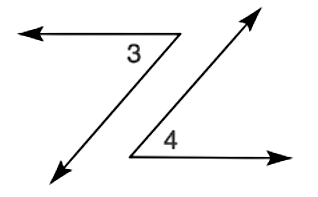Chapter P.3, Problem 26EElementary Geometry For College St...

7th Edition
Alexander + 2 others
ISBN: 9781337614085

Solutions

Chapter
SectionElementary Geometry For College St...

7th Edition
Alexander + 2 others
ISBN: 9781337614085
Textbook Problem

The sides of the pair of angles are parallel. Are ∠ 3 and ∠ 4 congruent?To determine

Whether 3 and 4 congruent.

Explanation

Calculation:

Given,

The sides of the pair of angles are parallel.

In the above figure, one of the side of each of the angle appears to be the transversal between the two other parallel side of those two angles...

Still sussing out bartleby?

Check out a sample textbook solution.

See a sample solution

The Solution to Your Study Problems

Bartleby provides explanations to thousands of textbook problems written by our experts, many with advanced degrees!

Get Started

In Exercises 23-36, find the domain of the function. 25. f(x)=3x+1x2

Applied Calculus for the Managerial, Life, and Social Sciences: A Brief Approach

21. If has the graph in Problem 5(a), find the following. (a) f(9) (b) f(5)

Mathematical Applications for the Management, Life, and Social Sciences

Convert the following percents to decimals. 35

Contemporary Mathematics for Business & Consumers

Find an expression for the mean of the probability density function .

Study Guide for Stewart's Single Variable Calculus: Early Transcendentals, 8th